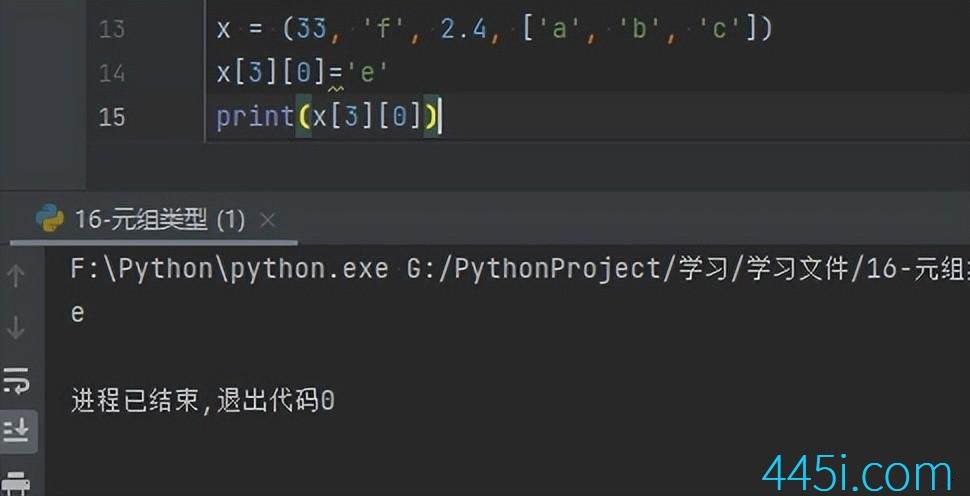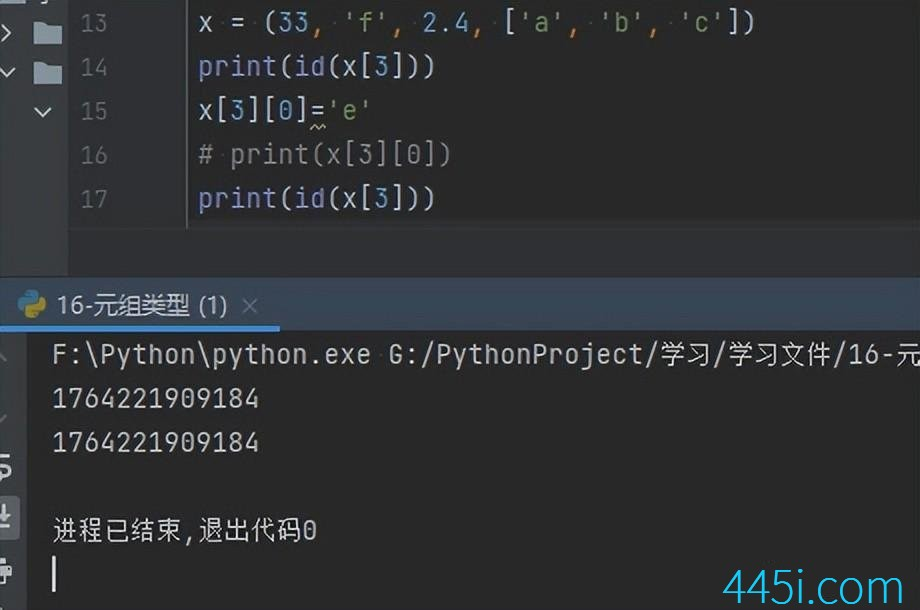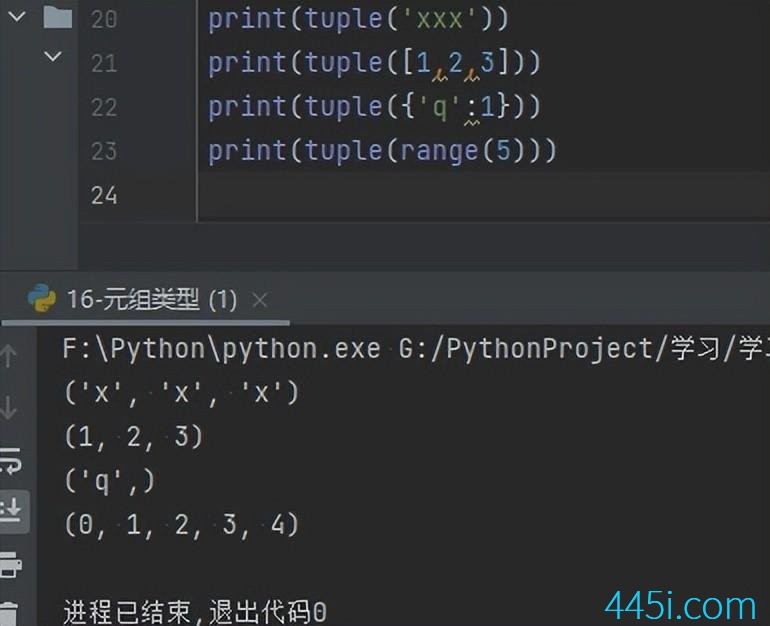# Python 元组类型2

``````x = (33, 'f', 2.4）
x=(x)
print(x)````````````x = (33, 'f', 2.4, ['a', 'b', 'c'])
x='e'
print(x)````````````x = (33, 'f', 2.4, ['a', 'b', 'c'])
print(id(x))
x='e'
print(id(x))````````````print(tuple('xxx'))
print(tuple([1,2,3]))
print(tuple({'q':1}))
print(tuple(range(5)))``````### 觉得文章有用就打赏一下文章作者

#### 支付宝扫一扫打赏#### 微信扫一扫打赏